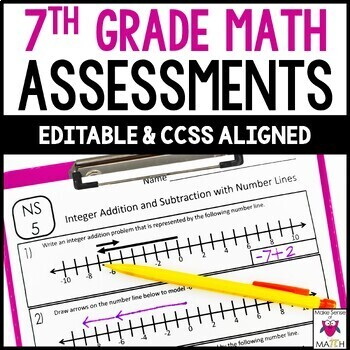# 7th Grade Math Assessments Common Core Bundle EDITABLE7th
Subjects
Standards
Resource Type
Formats Included
• Zip
Pages
65 pages
\$12.00
Bundle
List Price:
\$18.50
You Save:
\$6.50
\$12.00
Bundle
List Price:
\$18.50
You Save:
\$6.50

#### Products in this Bundle (7)

showing 1-5 of 7 products

#### Also included in

1. Looking to save time, money and sanity? Then this 7th grade math curriculum and activities bundle is for you! This curriculum is aligned to the 7th grade common core standards. Each unit includes: guided notes, assessments/worksheets and activities such as: task cards, stations, mystery pictures, w
\$250.00
\$403.00
Save \$153.00

### Description

Save yourself some times with these EDITABLE math assessments aligned to the 7th grade math common core. 30 assessments covering rational numbers, expressions, equations, inequalities, proportional reasoning, proportional relationships, geometry, statistics, and probability. Focus is placed on fluency AND clear understanding of concepts. Students will calculate, write, model, analyze and explain to show their understanding of the common core concepts.

By purchasing this Bundle you are saving over 30% off each individual product.

This product is EDITABLE!

• Includes a complete PDF document of assessments
• Includes a PowerPoint document that allows you to edit the questions
• You can create various versions to use in your math classroom

• Quick assessments
• Quizzes
• Reviews
• Interventions
• Homework
• Track student progress and mastery of concepts

Assessments are specifically aligned to 7th Grade CCSS

Rational Numbers

• Integer addition and subtraction fluency
• Integer multiplication and division fluency
• Integer addition and subtraction with number lines
• Rational number addition and subtraction fluency
• Rational number multiplication and division fluency
• Rational number understanding
• Rational number word problems

Algebra: Expressions, Equations & Inequalities

• Simplifying expressions fluency
• Applied expressions
• Solving equations fluency
• Word problems and equations
• Solving inequalities fluency
• Word problems and inequalities

Proportional Relationships

• Calculating Unit Rates
• Proportional Relationships
• Constant of Proportionality
• Proportional Graphs and Equations

Proportional Reasoning

• Ratios and Percent Problems
• Scale Drawings

Geometry

• Angle Basics
• Angles & Algebra
• Triangle Construction
• Circles: Circumference and Area Fluency
• Circles: Circumference and Area Understanding
• 2d Area
• 3d Surface Area and Volume
• 3d Slicing

Probability

• Probability
• Compound Events

Statistics

• Statistics

Also available for 8th Grade Math

"I am so thankful to have come across these! Well done and saves me a lot of time!"

"I am so glad I purchased this resource! The items included in this will be perfect to check for iep goals and make sure my kiddos are reaching proficiency in particular standards as well as to check in for retention. Thanks for creating this product!"

FOLLOW ME for more great products to make sense of math!

Remember that leaving feedback earns you points toward FREE TPT purchases!

Please feel free to contact me with any questions!

Michelle,

Make Sense of Math

Total Pages
65 pages
Included
Teaching Duration
N/A
Report this Resource to TpT
Reported resources will be reviewed by our team. Report this resource to let us know if this resource violates TpT’s content guidelines.

### Standards

to see state-specific standards (only available in the US).
Use proportional relationships to solve multistep ratio and percent problems. Examples: simple interest, tax, markups and markdowns, gratuities and commissions, fees, percent increase and decrease, percent error.
Explain what a point (𝘹, 𝘺) on the graph of a proportional relationship means in terms of the situation, with special attention to the points (0, 0) and (1, 𝘳) where 𝘳 is the unit rate.
Represent proportional relationships by equations. For example, if total cost 𝘵 is proportional to the number 𝘯 of items purchased at a constant price 𝘱, the relationship between the total cost and the number of items can be expressed as 𝘵 = 𝘱𝘯.
Identify the constant of proportionality (unit rate) in tables, graphs, equations, diagrams, and verbal descriptions of proportional relationships.
Decide whether two quantities are in a proportional relationship, e.g., by testing for equivalent ratios in a table or graphing on a coordinate plane and observing whether the graph is a straight line through the origin.